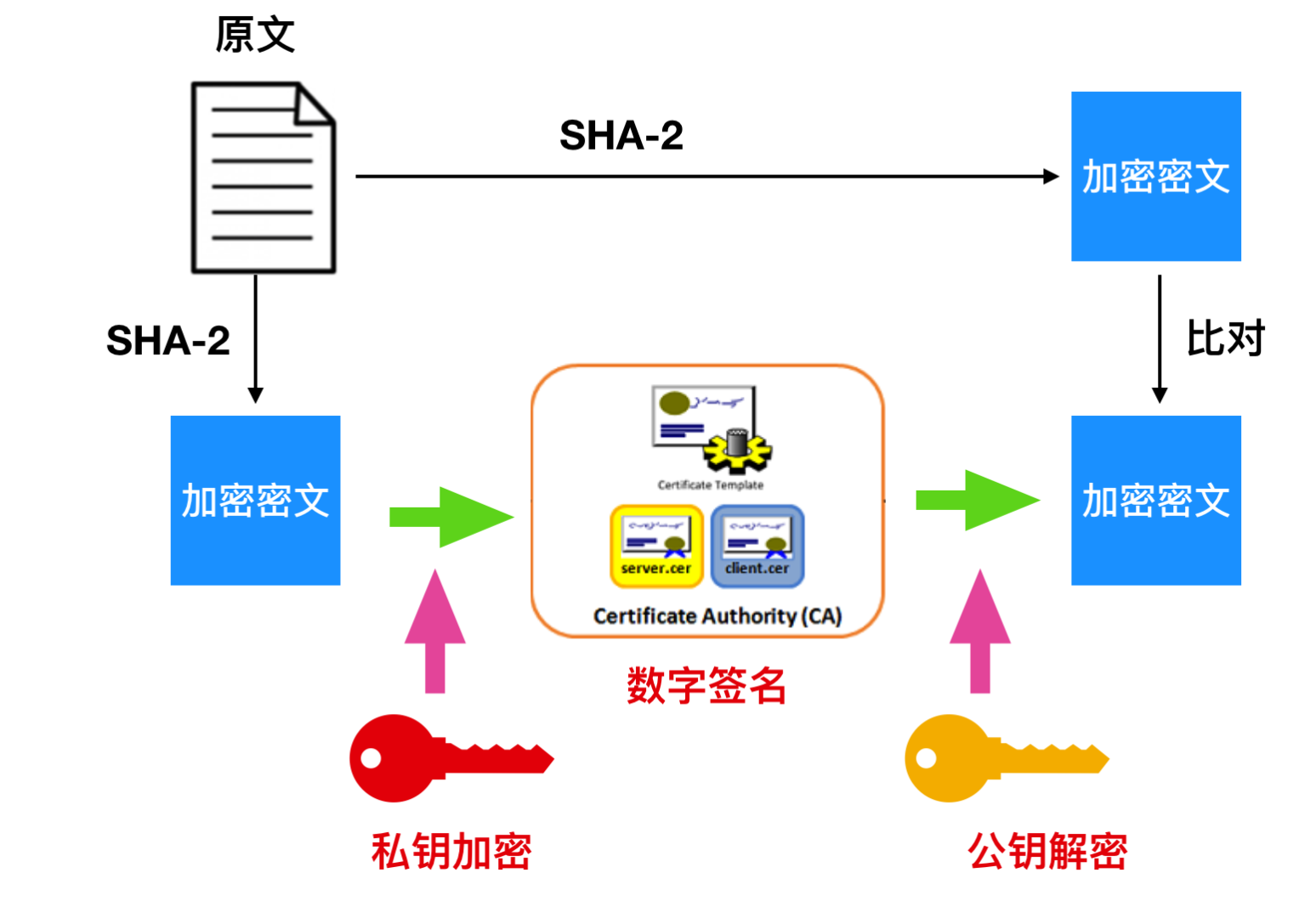# 面试刷题记录(持续更新中)

## 1.面试题:跳水板

shorter = 1
longer = 2
k = 3

0 < shorter <= longer
0 <= k <= 100000

### 题解：

然后考虑一般情况，即 shorter0k>0k>0。由于短木板和长木板一共使用 kkk 块，因此一共有 k+1k+1k+1 种组合，每种组合下建造的跳水板长度都是不一样的，一共有 k+1k+1k+1 种不同的长度。
为什么每种组合下建造的跳水板长度都是不一样的？考虑以下两种不同的组合：第一种组合，有 iii 块长木板，则跳水板的长度是 shorter∗(k−i)+longer∗ishorter(k-i)+longerishorter∗(k−i)+longer∗i；第二种组合，有 jjj 块长木板，则跳水板的长度是 shorter∗(k−j)+longer∗jshorter(k-j)+longerjshorter∗(k−j)+longer∗j。其中 0≤ishorterlonger>shorterlonger>shorter 且 i<ji<ji<j，因此上式的值小于 000。由此可见，任意两种不同的组合下的跳水板长度都是不一样的，而且使用的长木板越多，跳水板的长度越大。
因此创建长度为 k+1k+1k+1 的数组 lengths\text{lengths}lengths，对于 0≤i≤k0 \leq i \leq k0≤i≤k，令 lengths[i]=shorter∗(k−i)+longer∗i\text{lengths}[i]=shorter(k-i)+longerilengths[i]=shorter∗(k−i)+longer∗i，则 lengths\text{lengths}lengths 包含跳水板所有可能的长度，且长度为升序排序。

### JAVA解决方案

class Solution {
public int[] divingBoard(int shorter,int longer,int k) {
if (k == 0) {
return new int;
}
if (shorter == longer) {
return new int[]{shorter * k};
}
int[] lengths = new int[k + 1];
for (int i = 0; i <= k; i++) {
lengths[i] = shorter * (k - i) + longer * i;
}
return lengths;
}
}


## 2.面试题：恢复空格

输入：
dictionary = ["looked","just","like","her","brother"]
sentence = "jesslookedjustliketimherbrother"



0 <= len(sentence) <= 1000
dictionary中总字符数不超过 150000。



### 题解：

定义 dp[i]\textit{dp}[i]dp[i] 表示考虑前 iii 个字符最少的未识别的字符数量，从前往后计算 dp\textit{dp}dp 值。
考虑转移方程，每次转移的时候我们考虑第 j(j≤i)j(j\le i)j(j≤i) 个到第 iii 个字符组成的子串 sentence[j−1⋯i−1]\textit{sentence}[j-1\cdots i-1]sentence[j−1⋯i−1] （注意字符串下标从 000 开始）是否能在词典中找到，如果能找到的话按照定义转移方程即为：

dp[i]=min⁡(dp[i],dp[j−1])\textit{dp}[i]=\min(\textit{dp}[i],\textit{dp}[j-1]) dp[i]=min(dp[i],dp[j−1])

否则没有找到的话我们可以复用 dp[i−1]\textit{dp}[i-1]dp[i−1] 的状态再加上当前未被识别的第 iii 个字符，因此此时 dp\textit{dp}dp 值为

dp[i]=dp[i−1]+1\textit{dp}[i]=dp[i-1]+1 dp[i]=dp[i−1]+1

&nbsp最后问题化简成了转移的时候如何快速判断当前子串是否存在于词典中，与「单词拆分」类似我们可以选择用哈希表来优化，但笔者实测下来速度很慢，因为用哈希表来实现本身有两个问题，一个是哈希表本身的常数很大，还有一个是我们在枚举子串是否在词典中的时候有些其实是没有必要的枚举。简单举例，如果我们有词典：['aabc', 'babc', 'cbc'] ，但是我们在倒序枚举的时候检查 dc 这个子串没出现在词典中以后我们就没必要再接着往前枚举是否有合法的子串了，因为 dc 本身已经不是词典中「任意一个单词的后缀」，我们再接着枚举 dc 或者 *dc 判断其是否在词典中都是无用功。

因此最终笔者选择了用字典树 Trie\textit{Trie}Trie 来优化查找，Trie\textit{Trie}Trie 是一种最大程度利用多个字符串前缀信息的数据结构，它可以在 O(w)O(w)O(w) 的时间复杂度内判断一个字符串是否是一个字符串集合中某个字符串的前缀，其中 www 代表字符串的长度。这里具体实现不再展开，我们只讲怎么使用。上文提到了哈希表实现的时候会出现很多冗余的判断，最关键的一点就是当前枚举的子串已经不再是词典中「任意一个单词的后缀」，这点我们可以利用 Trie\textit{Trie}Trie 来解决。

我们将词典中所有的单词「反序」插入字典树中，然后每次转移的时候我们从当前的下标 iii 出发倒序遍历 i−1,i−2,⋯ ,0i-1,i-2,\cdots,0i−1,i−2,⋯,0。在 Trie\textit{Trie}Trie 上从根节点出发开始走，直到走到当前的字符 sentence[j]\textit{sentence}[j]sentence[j] 在 Trie\textit{Trie}Trie 上没有相应的位置，说明 sentence[j⋯i−1]\textit{sentence}[j\cdots i-1]sentence[j⋯i−1] 不存在在词典中，且它已经不是「任意一个单词的后缀」，此时我们直接跳出循环即可。否则，我们需要判断当前的子串是否是一个单词，这里我们直接在插入 Trie\textit{Trie}Trie 的时候在单词末尾的节点打上一个 isEnd\textit{isEnd}isEnd 的标记即可，这样我们在走到某个节点的时候就可以判断是否是一个单词的末尾并根据状态转移方程更新我们的 dp\textit{dp}dp 值。

### JAVA解决方案

class Solution {
public int respace(String[] dictionary, String sentence) {
int n = sentence.length();

Trie root = new Trie();
for (String word: dictionary) {
root.insert(word);
}

int[] dp = new int[n + 1];
Arrays.fill(dp, Integer.MAX_VALUE);
dp = 0;
for (int i = 1; i <= n; ++i) {
dp[i] = dp[i - 1] + 1;

Trie curPos = root;
for (int j = i; j >= 1; --j) {
int t = sentence.charAt(j - 1) - 'a';
if (curPos.next[t] == null) {
break;
} else if (curPos.next[t].isEnd) {
dp[i] = Math.min(dp[i], dp[j - 1]);
}
if (dp[i] == 0) {
break;
}
curPos = curPos.next[t];
}
}
return dp[n];
}
}

class Trie {
public Trie[] next;
public boolean isEnd;

public Trie() {
next = new Trie;
isEnd = false;
}

public void insert(String s) {
Trie curPos = this;

for (int i = s.length() - 1; i >= 0; --i) {
int t = s.charAt(i) - 'a';
if (curPos.next[t] == null) {
curPos.next[t] = new Trie();
}
curPos = curPos.next[t];
}
curPos.isEnd = true;
}
}


##### 热门推荐
•浏览(1,220)
•浏览(1,173)
•浏览(820)
•浏览(784)
•浏览(661)
•浏览(547)
•浏览(498)
•浏览(470)
•浏览(433)
•浏览(433)